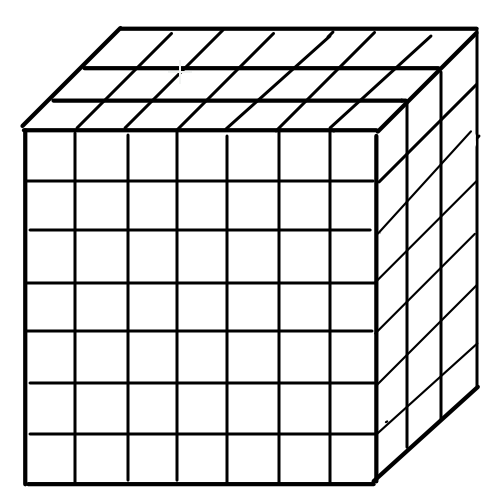A cuboid is of dimensions$60cm \times 54cm \times 30cm$. How many small cubes with side $6cm$can be placed in the given cuboid?Verified
142.5k+ views
Hint: This question is based on the concept of volume. According to the question,
The volume of cuboid$= n \times$ volume of small cubes, where $n$ is the number of small cubes. And we know that
Volume of cuboid$= l \times b \times h$, where $l$ is length,$b$is breadth and $h$ is height.
Volume of cube$= {a^3}$, where $a$ is length of any side.In this question, we have to find the number of cubes that can be placed in the cuboid. It means that the volume of the cuboid will be equal to the sum of the volume of all small cubes that can be placed in the cuboid. Therefore,
If $n$is the number of small cubes.
Volume of cuboid$= n \times$ volume of small cubes
$\Rightarrow l \times b \times h = n \times {a^3}$ [Here$l$, $b$ and $h$ are the length, breadth and height of the cuboid and $a$ is the side of the cube.]
It is given that,$l = 60cm$, $b = 54cm$, $h = 30cm$ and $a = 6cm$
On substituting the values,
$\therefore 60 \times 54 \times 30 = n \times {6^3}$
$\Rightarrow 97200 = n \times 216$
$\Rightarrow n = \dfrac{{97200}}{{216}}$
$\Rightarrow n = 450$
Hence there can be $450$ small cubes with side $6cm$ can be placed in the given cuboid.

Note:
To avoid the error one should change the unit of all the dimensions in the same unit then should start to calculate the answer.